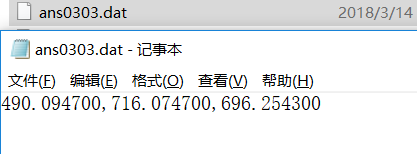0%

# Matplotlib 设置图片x轴文字方向

• 主要是plt.xticks中的rotation选项控制底部标签旋转。

## 正常方向标签画法（默认）

``````import matplotlib.pyplot as plt
import numpy as np
import pandas as pd

x=["第一个柱","第二个柱","第三个柱","第4个柱"]
y=[50,40,30,20]
plt.bar(x,y)
plt.show()
``````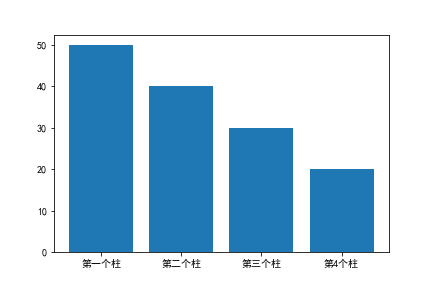## 45°标签画法

``````plt.bar(x,y)
plt.xticks(rotation="45")
plt.savefig("45旋转.png")
plt.show()
``````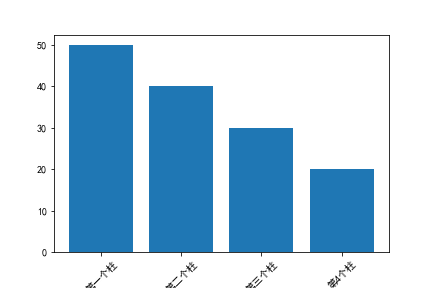## 其他角度

``````plt.figure(figsize=(5,5),dpi=300)
plt.bar(x,y)
plt.xticks(rotation="90",fontsize=20)
plt.savefig("90旋转.png")
plt.show()
``````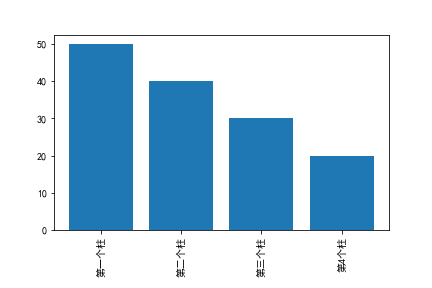# jupyter基本安装与内核安装

## 1. 安装jupyter

1.1 在python3安装jupyter

``````py -3 -m pip install jupyter
``````

## 2. 启动

2.1 在命令提示框输入

``````cd E:\jupyter    #一会jupyter在这个目录打开
jupyter notebook #可以指定端口
``````

## 3. 增加内核

3.1 安装ipykernel

``````py -3 -m pip install ipykernel
py -2 -m pip install ipykernel #2的这句会报错
``````

``````py -2 -m pip install ipykernel==8888 #先指定一个错误的版本，在返回的错误信息中会有版本列表。
py -2 -m pip install ipykernel==4.8.2 #指定上述列表版本内的一个版本
``````

``````py -2 -m ipykernel install --user
``````

## 运行结果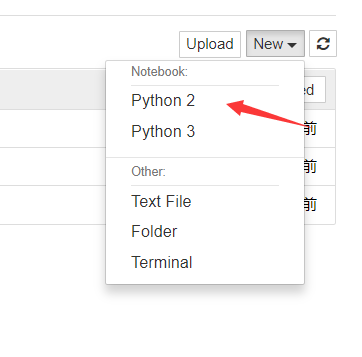## 2. 功能说明

1. 上传本地文件至OSS
2. OSS自动为图片加水印
3. 如果粘贴进去的是链接，则将图片下载后上传，并删除本地对应文件。

## 3. 所需库安装

``````pip install oss2   #用于图片的上传
pip install pyperclip   #用于MarkDown图片代码的复制
pip install requests   #获取远程图片
``````

## 4. 代码展示

``````#encoding:utf-8
import oss2
import os
import datetime
import pyperclip
import requests

filePath = str(input()).strip()   #去除链接/路径前后的空格
if filePath=="":
return

fileName = os.path.basename(filePath)   #提取文件名
if "https://" in filePath:   #判断是否为网络文件（是否需要下载）
r=requests.get(filePath)
with open(fileName,"ab") as tmp:
tmp.write(r.content)
filePath=fileName

now = datetime.datetime.now()
t = now.strftime("%Y_%m_%d_%H_%M_%S_")
remoteFileName = 'Markdown_image/' + str(t) + fileName  #设置远程文件名
result = bucket.put_object_from_file(remoteFileName, filePath)  #上传文件

if result.status == 200:
print("上传成功！")
pyperclip.copy(mkstyle)   #复制到剪贴板
print("已成功提交至oss,并成功复制")
os.remove(filePath)   #删除本地文件
else:
print(result.status)
print("网络出错，或出现其他未知原因！")

if __name__=="__main__":
AccessKeyID="123456"
AccessKeySeret="abcdef"
auth = oss2.Auth(AccessKeyID, AccessKeySeret) #认证
service = oss2.Service(auth=auth, endpoint='oss-cn-shanghai.aliyuncs.com')
bucket = oss2.Bucket(auth, 'oss-cn-shanghai.aliyuncs.com', "harrycodesitefile") #创建用来上传文件的的bucket
while(True):
print("准备拖动图片上传。")
``````

## 5. 运行展示

1. 准备上传图片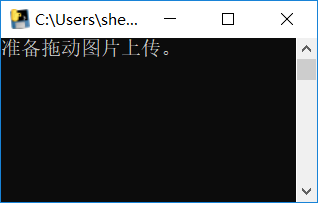2. 将图片直接拖至黑框中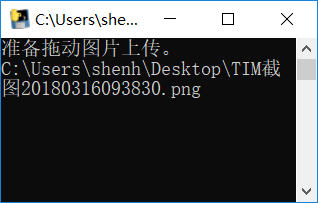3. 回车上传图片至OSS，并直接获取MarkDown语法的图片链接。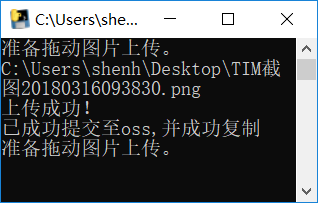## 6. 说明

• 本次写的图片上传工具，是通用的，不是上传至某个公共图床，为了自己网站图片不挂，还是自己保管最为妥当。对象存储可以去各大市场选购。

# 修改matplotlib以支持中文

## 1. 打开以下路径

``````C:\Python27\Lib\site-packages\matplotlib\mpl-data
``````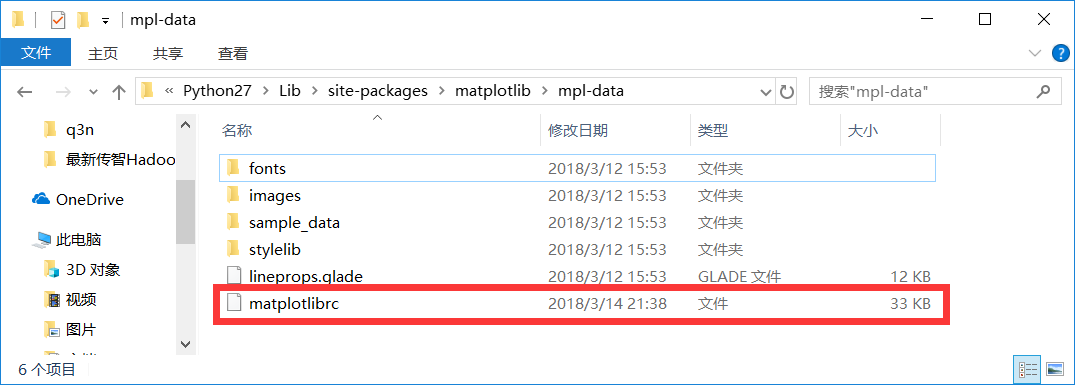修改上面图片中框出的文件。

## 3. 修改文件

1. 首先去掉`font.family`前面用于注释的“#”号。
2. 再去掉`font.sans-serif`前面的“#”号。
3. `font.sans-serif`那一行添加本机存在的中文字体。（在此用黑体演示，一般电脑都存在黑体。）
4. axes.unicode_minus删去前面的#，并修改为False
5. 保存当前文档。

# 数据分析练习（2018）

• 数据为自己生成的练习数据

``````电影：A:大世界、B:捉妖记2、C:红海行动

``````

## Q1

1. 编程统计并输出影片A的上映天数和日平均票房（日平均票房指文件中的所有涉及城市总平 均票房），程序源代码保存成ans0301.py，并将结果保存于ans0301.dat，要求ans0301.dat只包 含1个long型数据和一个1个浮点型数据，浮点数据以万元为单位，保留6位小数，2个数以英文逗 号分隔，不换行，文件样例如下：

123, 23.123456

#### 解题过程：

``````#encoding:utf-8
import numpy as np
import pandas as pd

if __name__=="__main__":
name=["time","filmName","place","dyy","pf"]  #列名
days=len(data[data.filmName=="大世界"].time.unique())  #获取大世界这本电影的上映的日期（去重）
average_money=data[data.filmName=="大世界"].sum()["pf"]/float(days) #求出大世界这部电影的日平均票房（单位：元）
average_money=average_money/10000.0  #将单位元转化为万元
result="{},{:.6f}".format(days,average_money)  #准备插入的文本
f=open("ans0301.dat","w")  #新建文件
f.write(result)   #写入准备的字符串
f.close()
``````
##### 生成文件效果图：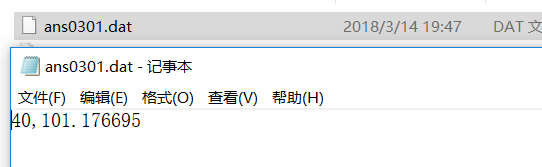## Q2

2、利用Bar函数编程输出影片A、B、C的周平均票房（周平均票房指文件中的所有涉及城市周 票房总平均），Y轴表示票房收入，单位万元；X轴表示电影名称，电影名称的排列从左至右以A、 B、C为准，要求将输出的直方图保存成图像文件ans0302.jpg，程序源代码保存成ans0302.py， 另外，将三部电影各自的票房总收入按自高到低的顺序存入ans0302.dat文件中，要求 ans0302.dat中只包含3个浮点型票房数据，以万元为单位，保留6位小数，数据以英文逗号分隔， 不换行，文件样例如下：

``````23.123456,20.654321,18.123456
``````

#### 解题过程：

``````#encoding:utf-8
import numpy as np
import pandas as pd
import matplotlib.pyplot as plt

def get_weeks(days):
weeks_tmp=days // 7
if days % 7 != 0:
weeks = weeks_tmp + 1
return weeks*1.0
return weeks_tmp*1.0

if __name__=="__main__":
name = ["time", "filmName", "place", "dyy", "pf"]
# A电影的周平均票房
days_A = len(data[data.filmName=="大世界"].time.unique())
zpf_A = data[(data.filmName == "大世界")].sum()["pf"]
weekpf_A = zpf_A / get_weeks(days_A)
weekpf_A=weekpf_A/10000.0
# B电影的周平均票房
days_B = len(data[data.filmName=="捉妖记2"].time.unique())
zpf_B = data[data.filmName == "捉妖记2"].sum()["pf"]
weekpf_B = zpf_B / get_weeks(days_B)
weekpf_B=weekpf_B/10000.0
# C电影的周平均票房
days_C = len(data[data.filmName=="红海行动"].time.unique())
zpf_C = data[data.filmName == "红海行动"].sum()["pf"]
weekpf_C = zpf_C / get_weeks(days_C)
weekpf_C=weekpf_C/10000.0

dy_names = ["大世界", "捉妖记2", "红海行动"]
dy_data = [weekpf_A, weekpf_B, weekpf_C]
# A画图并保存
plt.bar(dy_names, dy_data)
plt.savefig("ans0302.jpg")  #此处需要安装pillow，如果没有安装，保存成jpg会直接报错。
#可以先保存成png，然后用画图工具保存成jpg
dy_data=sorted(dy_data,reverse=True)
# 将结果写入文件
result_string = "%.6f,%.6f,%.6f"%tuple(dy_data)
print result_string
f = open("ans0302.dat", "w")
f.write(result_string)
f.close()
``````
##### 绘制的图片：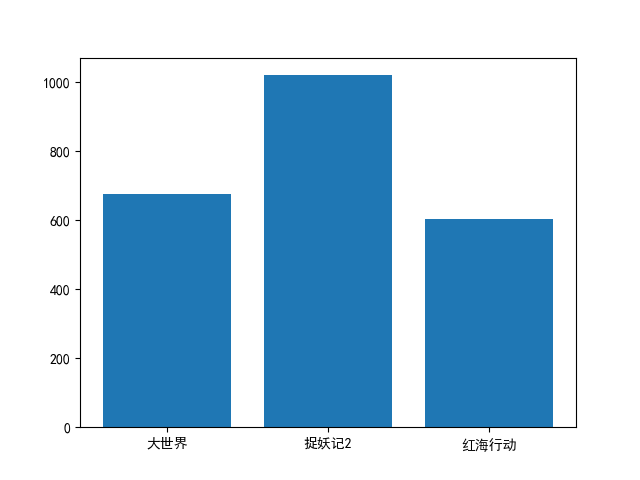注，因为暂时没有修改matplotlib的配置文件支持中文。

##### ans0302.dat数据展示：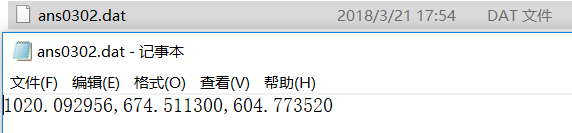## Q3

3、编程，利用折线图，画出影片A、B、C各自的周票房（周票房指文件中的所有涉及城市总周 票房）收入变化，要求将输出的折线图保存成图像文件ans0303.jpg，程序源代码保存成 ans0303.py，Y轴表示票房收入，单位为“万元”；X轴表示时间，以“0、1、2、3…n”的非负 整数作为刻度值，单位为“周”，要求： 1）折线图中含图例； 2）三部电影用不同的颜色和线型表达； 3）将电影A第一周的票房收入，电影B第二周的票房收入，电影C第三周的票房收入顺序存入 ans0303.dat文件中，注意ans0303.dat只包含3个浮点型票房数据，以万元为单位，保留6位小数，数据以英文逗号分隔，不换行，文件样例如下：

``````23.123456,20.654321,18.123456
``````

4）对本题周票房的说明如下：若某部电影从某月2日开始上映，则从当月2日到8日为其第一周 票房，9日至15日为其第2周票房，以此类推.

#### 解答过程：

``````#encoding:utf-8
import numpy as np
import pandas as pd
import matplotlib.pyplot as plt

name = ["time", "filmName", "place", "dyy", "pf"]
data.time=data.time.apply(lambda x:pd.to_datetime(x,format="%Y-%m-%d"))
data["weekofyear"]=data.time.apply(lambda x:x.weekofyear)
filmlist=["大世界","捉妖记2","红海行动"]
tmp_A=data[data.filmName=="大世界"].groupby(["weekofyear"]).sum()/10000.0
tmp_B=data[data.filmName=="捉妖记2"].groupby(["weekofyear"]).sum()/10000.0
tmp_C=data[data.filmName=="红海行动"].groupby(["weekofyear"]).sum()/10000.0

tmp_A.reset_index(inplace=True)
tmp_B.reset_index(inplace=True)
tmp_C.reset_index(inplace=True)

A1=np.array(tmp_A)
B1=np.array(tmp_B)
C1=np.array(tmp_C)

l1,=plt.plot(tmp_A.index,tmp_A.pf,ls="-")
l2,=plt.plot(tmp_B.index,tmp_B.pf,ls="-.")
l3,=plt.plot(tmp_C.index,tmp_C.pf,ls=":")
plt.legend(handles=[l1,l2,l3],labels=[u"电影A",u"电影B",u"电影C"])
plt.xlabel("weeks")
plt.ylabel("Money")
plt.savefig("ans0303.jpg")
# #此处需要安装pillow，如果没有安装，保存成jpg会直接报错。
# #可以先保存成png，然后用画图工具保存成jpg
plt.show()

result_string="{:.6f},{:.6f},{:.6f}".format(A1,B1,C1)
f=open("ans0303.dat","w")
f.write(result_string)
f.close()
``````
##### 绘制的图片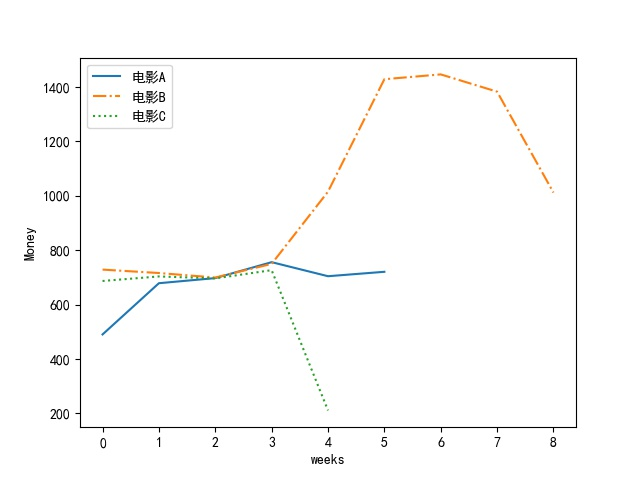注：因为数据为生成的，图片有些许奇怪。

##### ans0303.dat内容展示：